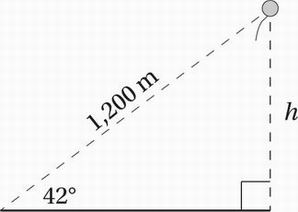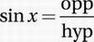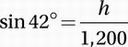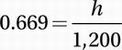# SAT Math Multiple Choice Question 697: Answer and Explanation

### Test Information

Question: 697

7.Alyssa determines that a floating balloon is 1,200 meters away from her at an angle of 42° from the ground, as in the figure above. What is the height, h, of the balloon from the ground? (sin 42° = 0.669, cos 42° = 0.743, tan 42° = 0.900)

• A. 802.8 meters
• B. 891.6 meters
• C. 1,080 meters
• D. 1,793 meters

Explanation:

A

Remember the definitions of the basic trigonometric functions: SOH CAH TOA. Since the "side of interest" (h) is the opposite side to the given angle (42°), and since we know the length of the hypotenuse (1,200), we should use SOH.Plug in the values:Substitute sin 42° = 0.669:Multiply by 1,002:

(1,200)(0.669) = 802.8 = h